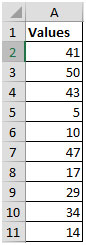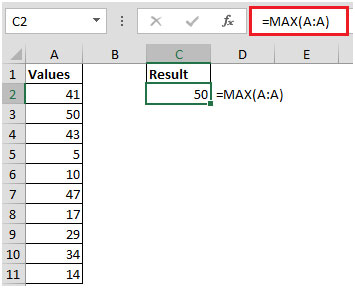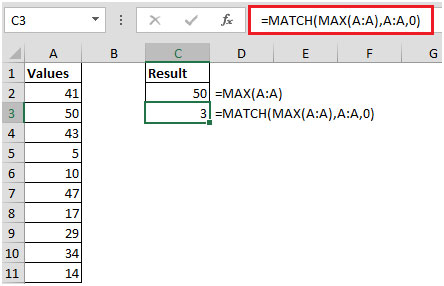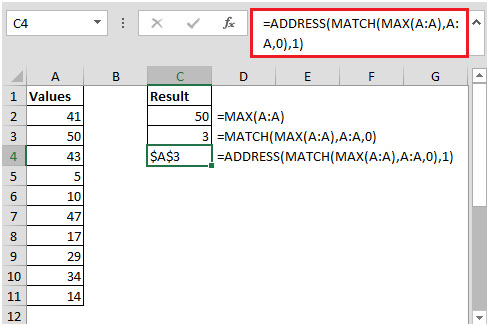# How To Find Cell Address Of The Maximum Value In A Column In Microsoft Excel

In this article, you will learn how to find address of the cell containing maximum value in a column.

We can use MAX, MATCH & ADDRESS functions to get the output.

Let us understand with examples:

• We have some random numbers in column A• Based on the above list, we can find out the maximum value using MAX function
• In cell C2, enter the function as =MAX(A:A)• The next step is to use MATCH function with MAX
• In cell C3, the formula will be =MATCH(MAX(A:A),A:A,0), and hence,we get 3 as the output.• Here, output retrieved from MAX function will be considered as the lookup value for MATCH function.
• The last step is to combine the ADDRESS, MATCH & MAX functions to retrieve the result.
• In cell C4, the formula will be =ADDRESS(MATCH(MAX(A:A),A:A,0),1)• The first argument specifies the row number (combination of MATCH & MAX functions). The second argument specifies the column number.

In this way, you can get the address of the cell containing maximum value. This article can be helpful while working on large amount of data.

Terms and Conditions of use

The applications/code on this site are distributed as is and without warranties or liability. In no event shall the owner of the copyrights, or the authors of the applications/code be liable for any loss of profit, any problems or any damage resulting from the use or evaluation of the applications/code.